## General Question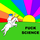# What number is a different random number every day?

Asked by Vincentt (8079) September 11th, 2013

I have a list containing the numbers between, say, 1 and 9. Now, I need an algorithm that randomly generates one of these numbers—but at any moment during the day it should produce the same number.

To clarify: let’s say that if I ran this algorithm this morning and it produced the number 3, then if my neighbour ran the same algorithm this evening, it should produce the number 3 as well. Likewise, if we had both ran it tomorrow, it should be likely to produce a different number than 3, but it should produce it twice.

It feels like a simple problem, but I can’t find a good answer…

Observing members: 0Composing members: 0So here’s the algorithm I’m using now:

1. Take the number of days since a certain day (e.g. 1 January 1970), so at September 11 2013 it is 15960).
2. Divide that number by the amount of possible numbers (which is 9, so 15960/9=1773.33333…).
3. The remainder is the number I use, so in this case 3.

The problem, however, is that tomorrow this algorithm will produce a 4, the day after that a 5, etc. until 9 where it will start at 1 again. I want the numbers to not be that easily predictable.

Vincentt (8079)“Great Answer” (0) Flag as…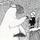Sounds like you want a random number generator based on your computer’s clock. Why involve dates?

glacial (12145)“Great Answer” (0) Flag as…I don’t want the generator to be completely random, merely chaotic using the present day as a seed.

So oops, bad wording. The reason I use date is because those are involved in the specific use case for this algorithm.

Vincentt (8079)“Great Answer” (0) Flag as…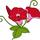One is the loneliest number

Coloma (47165)“Great Answer” (1) Flag as…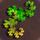What you want is a pseudo-random number generator seeded using the day’s date. As long as you are not planning on using this for anything super-important (like cryptography or statistics), then the PRNG can be very simple.

1) Take today’s date, written as a number. For example, today can be written as 91113, or even 9112013.

2) Square the number. 91113^2 = 8301578769

3) Remove the last (least) digit. Then remove the first digit. Repeat until there is only one digit.
8301 5 78769

4) The random number is a 5.

PhiNotPi (12680)“Great Answer” (6) Flag as…@PhiNotPi ‘s method will probably be sufficient. Most programming languages include pseudorandom number generators. You could, for example, download Python for free and fairly easily write code to generate pseudorandom numbers using the number of the day as a seed.

LostInParadise (29910)“Great Answer” (0) Flag as…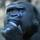I agree with the above advice. It would really help to know what you have in mind because, if this is being used as a shared secret for sending secure messages, or some other form, then it will be easily defeated.

gorillapaws (28894)“Great Answer” (0) Flag as…@PhiNotPi That’s great and makes a lot of sense, thanks!

And don’t worry, this has nothing to do with cryptography or anything, it’s just a method of showing a different recipe on my website every day.

Vincentt (8079)“Great Answer” (0) Flag as…Btw, on addition I had to do: the algorithm you mentioned above does not work with a variable amount of numbers, so I modified it to only remove the first and the last digit (so the number would be higher than the amount of numbers), and then take the remainder of dividing the resulting number with the amount of numbers. Works great now!

Vincentt (8079)“Great Answer” (0) Flag as…@Vincentt I thought that you only wanted a single digit. Anyways, the modification should be sufficiently random.

PhiNotPi (12680)“Great Answer” (0) Flag as…or